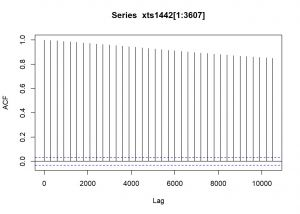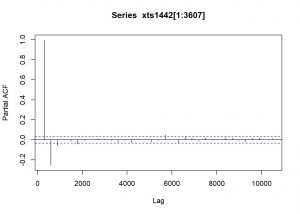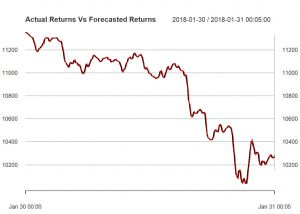# UNWE Article – Crypto Datathon

Prediction of cryptocurrency prices (5 min period) with AR, ARIMA and Neural Network models using R and Python.

8

Prediction of top 50 coins:

30-01-2018: forecast_1;

06-02-2018: forecast_2;

20-02-2018: forecast_3

09-03-2018: forecast_4

18-03-2018: forecast_5

MODELLING

• ARIMA MODELLING IN R
``````setwd("D:/DATA SCIENSE/Datathon/Academia Datathon/DATA")

# data20\$price_1442
# saving Time as POSIXct
# names(data20)
# str(data20)
data20\$time <- as.POSIXct(data20\$time)``````
``````#bitcoin 1442 separating
data1442 <- data20[,c(1,2)]

str(data1442)``````
``````## 'data.frame':    18752 obs. of  2 variables:
##  \$ time      : POSIXct, format: "2018-01-17 11:25:00" "2018-01-17 11:30:00" ...
##  \$ price_1442: num  10756 10788 10808 10776 10776 ...``````
``````#CREATE XTS OBJECT for 1442
# str(data1442\$time)
xts1442 <- xts(data1442[,-1], order.by = data1442[,1], frequency = 288)
xts1442[(1:6),]``````
``````##                        [,1]
## 2018-01-17 11:25:00 10756.0
## 2018-01-17 11:30:00 10788.1
## 2018-01-17 11:35:00 10807.5
## 2018-01-17 11:40:00 10776.1
## 2018-01-17 11:45:00 10776.1
## 2018-01-17 11:50:00 10729.7``````
``````#ACF and PACF test
acf(xts1442[1:3607])````````pacf(xts1442[1:3607])````````# FITTING auto.arima on the subset before 30-01-2018
ar1442<- auto.arima(xts1442[1:3607])
ar1442``````
``````## Series: xts1442[1:3607]
## ARIMA(1,1,1)
##
## Coefficients:
##          ar1      ma1
##       0.4451  -0.1809
## s.e.  0.0505   0.0554
##
## sigma^2 estimated as 1391:  log likelihood=-18165.28
## AIC=36336.56   AICc=36336.56   BIC=36355.13``````
``summary(ar1442)``
``````## Series: xts1442[1:3607]
## ARIMA(1,1,1)
##
## Coefficients:
##          ar1      ma1
##       0.4451  -0.1809
## s.e.  0.0505   0.0554
##
## sigma^2 estimated as 1391:  log likelihood=-18165.28
## AIC=36336.56   AICc=36336.56   BIC=36355.13
##
## Training set error measures:
##                     ME     RMSE      MAE          MPE      MAPE
## Training set 0.1141057 37.27926 22.49191 0.0007416239 0.1989633
##                     MASE         ACF1
## Training set 0.001967522 -0.001305298``````

auto.arima suggest ARIMA(1,1,1) which will be used to predict the prices in the below loop.

ARIMA FORECAST

``````# Initialzing an xts object for Actual Price
Actual_series = xts(0,as.POSIXct("2018-01-17 11:25:00","%Y-%m-%d %H:%M", tz = "EET"))

# Split the dataset in two parts - training and testing (for 30.01.2018 00:00:00)
breakpoint = floor(3608)
breakpoint``````
``##  3608``
``````# Initialzing a dataframe for the forecasted return series
forecasted_series = data.frame(Forecasted = numeric())

for (b in breakpoint:(3897)-1) {

coin1442_train = xts1442[1:b, ]
coin1442_test = xts1442[(b+1):nrow(xts1442), ]

# Summary of the ARIMA model using the determined (p,d,q) parameters from auto.arima fitted model
fit = arima(coin1442_train, order = c(1, 1, 1), include.mean=FALSE)
# summary(fit)

# Forecasting the price of BITCOIN
arima.forecast = forecast(fit, h = 1,level=99)

# Creating a series of forecasted price for the forecasted period
forecasted_series = rbind(forecasted_series,arima.forecast\$mean)
colnames(forecasted_series) = c("Forecasted")

# Creating a series of actual price for the forecasted period
Actual_return = xts1442[(b+1),]
Actual_series = c(Actual_series,xts(Actual_return))
rm(Actual_return)
options(warn = -1)

}

#CHECKING THE MODEL
Actual_series = Actual_series[-1]

# Create a time series object of the forecasted series
forecasted_series = xts(forecasted_series,index(Actual_series))

# Create a plot of the two return series - Actual versus Forecasted
plot(Actual_series,type='l',main='Actual Returns Vs Forecasted Returns')``````
``````lines(forecasted_series,lwd=1.5,col='red')
legend('bottomright',c("Actual","Forecasted"),lty=c(1,1),lwd=c(1.5,1.5),col=c('black','red'))````````````# Create a table for the accuracy of the forecast
comparsion = merge(Actual_series,forecasted_series)
comparsion\$Diff <- abs(comparsion\$Actual_series - comparsion\$Forecasted)
``````##                     Actual_series Forecasted       Diff
## 2018-01-30 00:00:00       11353.3   11349.66  3.6404400
## 2018-01-30 00:05:00       11350.7   11354.69  3.9887328
## 2018-01-30 00:10:00       11344.1   11350.26  6.1644392
## 2018-01-30 00:15:00       11338.0   11342.28  4.2777446
## 2018-01-30 00:20:00       11332.2   11336.06  3.8589676
## 2018-01-30 00:25:00       11321.4   11330.32  8.9166930
## 2018-01-30 00:30:00       11316.6   11318.21  1.6061508
## 2018-01-30 00:35:00       11311.2   11314.75  3.5540945
## 2018-01-30 00:40:00       11308.8   11309.44  0.6393984
## 2018-01-30 00:45:00       11288.3   11307.85 19.5473837``````
``````MAPE <- sum(comparsion\$Diff/comparsion\$Actual_series)*100/length(comparsion\$Actual_series)
MAPE``````
``##  0.1308932``

MAPE is 0.13 which is better than the shifted with lag 1 prices (0.15)

PREDICTION BASELINE in Python

We dumped the price of btc for each time point and “predict” that the next price at t+1 will be the same as the price at t. The MAPE for bitcoin price is 0.156%.

from numpy import mean
from sklearn.metrics import mean_squared_error, mean_absolute_error
from pandas import Series

data_dir = ‘/home/user/projects/crypto/20180427_hackaton/data/clean/’

series = Series.from_csv(data_dir + ‘btc.csv’, header=0)
# prepare data
X = series.values
X = X.astype(‘float32’)

# walk-forward validation
history = [x for x in X]
predictions = [history]
for i in range(1, len(X)):
prediction = history[i – 1]
predictions.append(prediction)

mae = mean_absolute_error(X, predictions)
print(‘MAE: %.3f’ % mae)

import numpy as np
def mape(y_true, y_pred):
return np.mean(np.abs((y_true – y_pred) / y_true)) * 100

print(“MAPE:”, mape(X, predictions))

• Autoregression in R

rm(list=ls())
setwd(“C:/Users/user/Desktop/R/data”)

#source(‘C:/Users/user/Desktop/R/data/hnkl2.R’)
#source(‘C:/Users/user/Desktop/R/data/hnkl.R’)
# Import data

n <- 6 # number of lags
sls <- 0.05 # max p-value

models = list()

m <- ncol(data)
s <- 2#77
for(j in 1:m){
y0 <- data[[s]]
name <- colnames(data)[s]

N <- length(y0)

y <- y0[(n + 1):N]
Ny <- length(y)
xx <- matrix(0, Ny, n)
for(i in 1:n) xx[,i] <- y0[(n – i + 1):(N – i)]
dat <- data.frame(y = y, xx)

datd <- dat[1:round(Ny/2),]
datv <- dat[(round(Ny/2) + 1):Ny,]

iterate <- 1
while(iterate){
model <- lm(y ~ . -1, datd)
pp <- anova(model)[,’Pr(>F)’]
pvalue <- pp[1:(length(pp) – 1)]
# print(anova(model))
if(any(pvalue > sls)){
ind <- which.max(pvalue)
xx <- xx[,-ind]
dat <- data.frame(y = y, xx)
datd <- dat[1:round(Ny/2),]
datv <- dat[(round(Ny/2) + 1):Ny,]
}else{
iterate <- 0
}
}

print(summary(model))
ym <- predict(model, datd)
yd <- datd\$y
ym <- predict(model, datv)
yv <- datv\$y
print(paste0(‘Ra2_dev = ‘, Ra2d))
print(paste0(‘Ra2_val = ‘, Ra2v))

Nv <- length(yv)
N0 <- 1
Nn <- Nv
plot(yv[N0:Nn], type = ‘l’)
title(name)
lines(ym[N0:Nn], col = 2)

models[[colnames(data)[s]]] <- model
s <- s + 3
}

# For the jury

Your_crypto <- ‘price_1442’
n <- 5005
your_data_points <- data[(n-5):n, Your_crypto]

y_next_moment <- predict(models[[Your_crypto]], y=your_data_points)[n+1]

#### 4 thoughts on “UNWE Article – Crypto Datathon”

1.metodinikolov says:
0

It would have been great if you had used what you learned in the first half about log-returns and how they behave in the prediction’s second half. As it stands it seems that you are using the prices to do the prediction, rather than any modification of it.
There also seem to be several different types of predictions – ARIMA(1, 1, 1) (which some what handles prices vs log-returns) and AR(1) model (which is an ARIMA(1, 0, 0)) – correct me if i am wrong, but you haven’t done any comparisons between the two models? As they are nested, readily available statistical tests could have been used for that part. Also, the final predictions are from which model?

1.martinmarinov says:
0

The AR(1) seemed to perform the best. Using an ARIMA doesn’t improve things much and it’s not surprising considering what we saw from the autocorrelation plots. The values just aren’t strongly correlated with one another, meaning a higher order model brings very little improvement, if any.

2.blessed123334 says:
0

3.archie says: